### Day 14 - Polynomials - 01.27.15

• Remediation/Retesting Protocol
• Unit 2 Test on Friday, 2/6!

Bell Ringer Quiz

1. Simplify the following: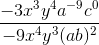1.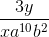2.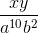3.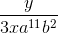4. none of the above

2. Solve for x in the following equation: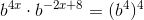1. 0

2. 2

3. 4

4. -2

5. none of the above

3. Solve for x in the following equation: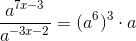1. 0

2. 1

3. 2

4. -2

5. none of the above

Review
• Prerequisites
• Exponents
• Relationship to multiplication
• Factors
• Reciprocal
• Monomials
• Multiplying Monomials
• Dividing Monomials

Lesson

Exit Ticket
• Posted on board at the end of the block
Lesson Objective(s)
• How are monomials related to polynomials?
• How is the degree of a polynomial found?

#### In-Class Help Requests

Standard(s)
• CC.9-12.A.APR.1 Perform arithmetic operations on polynomials. Understand that polynomials form a system analogous to the integers, namely, they are closed under the operations of addition, subtraction, and multiplication; add, subtract, and multiply polynomials.
• CC.6.EE.1 Apply and extend previous understandings of arithmetic to algebraic expressions.  Write and evaluate numerical expressions involving whole-number exponents.
• CC.9-12.N.RN.1 Extend the properties of exponents to rational exponents. Explain how the definition of the meaning of rational exponents follows from extending the properties of integer exponents to those values, allowing for a notation for radicals in terms of rational exponents. For example, we define 5^(1/3) to be the cube root of 5 because we want [5^(1/3)]^3 = 5^[(1/3) x 3] to hold, so [5^(1/3)]^3 must equal 5.

Mathematical Practice(s)
• #1 - Make sense of problems and persevere in solving them
• #2 - Reason abstractly and quantitatively
• #7 - Look for and make use of structure

Past Checkpoints